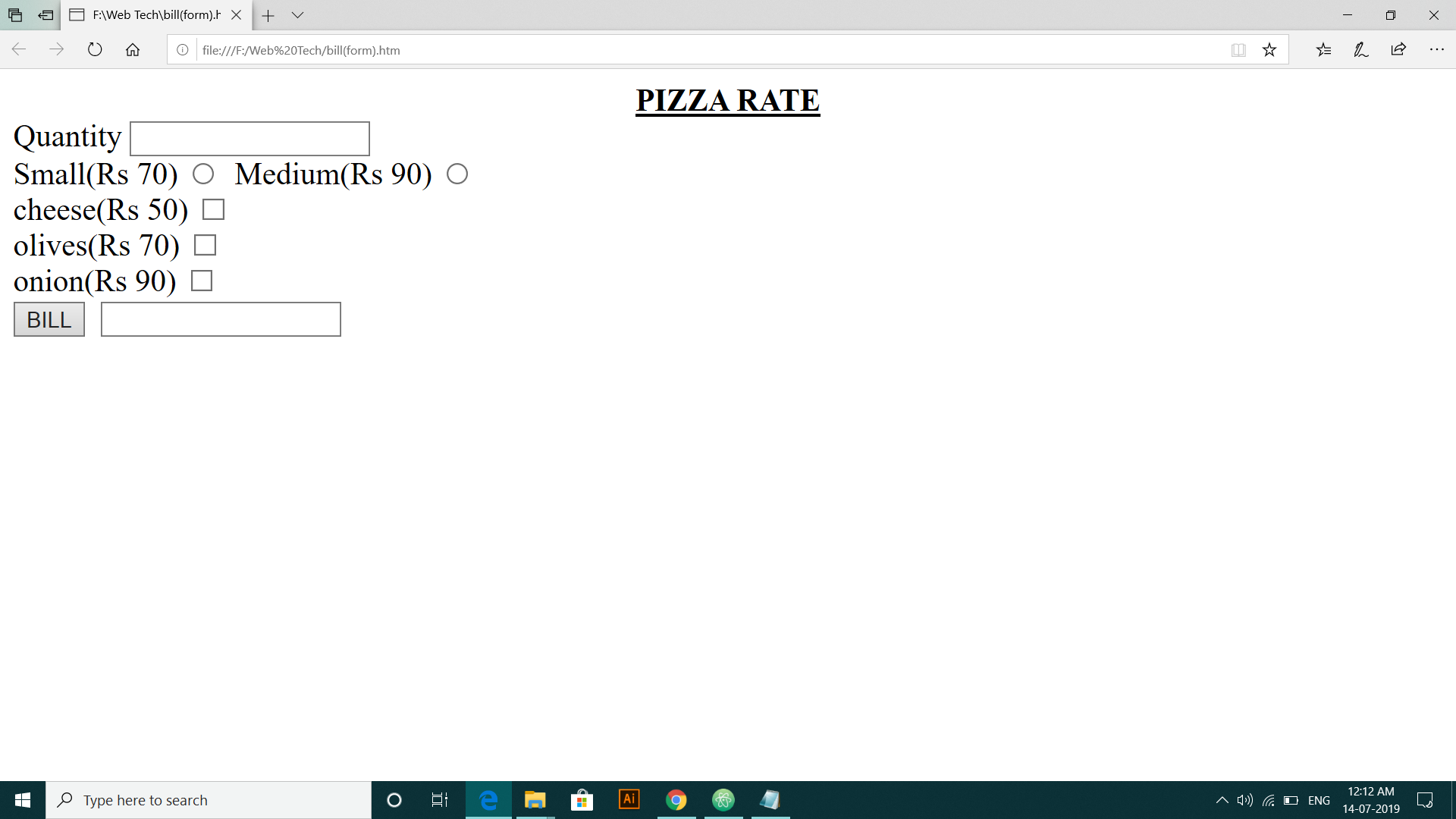# How to create a billing system for pizza using JavaScript

Hey Folks,
I am up with another tutorial of JavaScript. In this tutorial, we will learn how to create a billing system for pizza using JavaScript. You must have filled a form while ordering pizza I guess.

So almost every food website or app has such forms and one has filled it while ordering the food like what quantity you want to order and various add ons option is also there. And various checkboxes and buttons are there to ease our order.

## Code to create a billing system for pizza using JavaScript

```<script>
function ch()
{
var x,y
x=""
y=""
x=parseInt(x)
y=parseInt(y)
y=document.frm.t2.value
if(document.frm.r(0).checked)
x=70
if(document.frm.r(1).checked)
x=90
if(document.frm.c1.checked)
x=50+x
if(document.frm.c2.checked)
x=70+x
if(document.frm.c3.checked)
x=90+x
document.frm.t1.value=(x)*y
}
</script>
<form name="frm"><font text="cyan">
<center><big><b><u>PIZZA RATE</b></u></center>
</font>Quantity
<input type="text" name="t2">
<br>Small(Rs 70)
&nbsp;Medium(Rs 90)
<br>cheese(Rs 50)
<input type="checkbox" name="c1">
<br>olives(Rs 70)
<input type="checkbox" name="c2">
<br>onion(Rs 90)
<input type="checkbox" name="c3"><br>
<input type="button" value="BILL" onClick="ch()">
&nbsp;<input type="text" name="t1">
</form>```

We have used the basic framework of JavaScript which says that all the coding or mathematical part is done under script tag. But all the code related to display on our web browser is written under the form tag.

So the first thing is to select among the two options whether large size or small. So while writing in the code we use the value stored in document.frm.r(0) and r(1). And it means if the first radio button is selected so r(0).checked is true and vice-versa.We also check the value stored in checkboxes in the same way as of the radio button by document.frm.c1.checked. And we have an option like if you want to add cheese, olives, onion. You can also add all the ingredients at the same time as they are checkboxes.

ParseInt is used to predefine the data stored in a variable as an integer value. In the script part, we just keep adding the amount to our variable x and at last, we multiply it by our required quantity to get the total payable amount.

I hope you understood the code well. Feel free to ask your doubts in the comment section below.